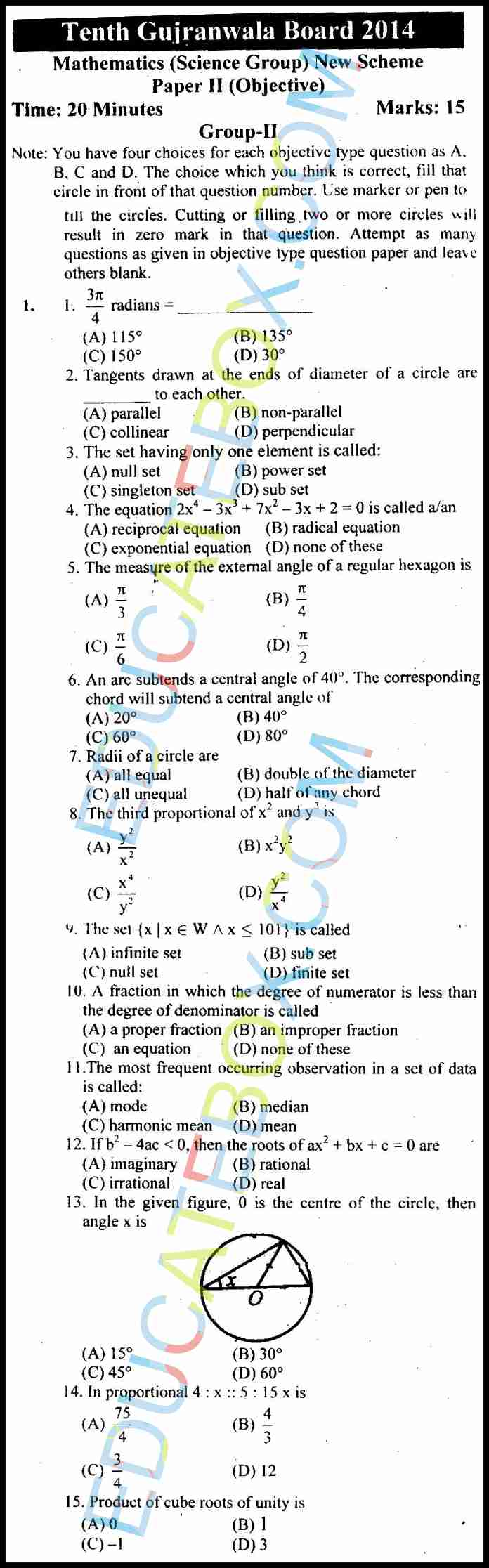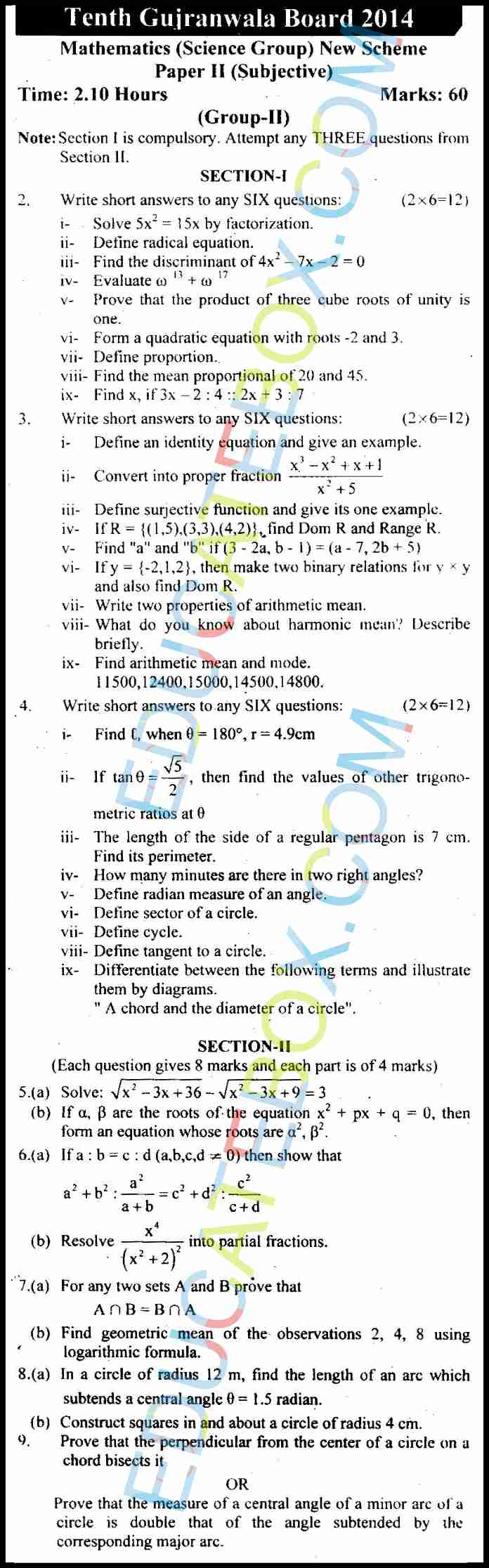# Past Paper 10th Maths (Science Group) Gujranwala 2014 Group2 (English Medium)

0
107

## Past Paper Class 10 Maths (Science Group) Gujranwala Board 2014 OBJECTIVE Type Group 2 (English Medium)

Some of the questions of this paper are as follows:

• The set having only one element is called?
• What is measure of the external angle of regular hexagon?
• What are Radii of a circle?
• A fraction in which the degree of numerator is less than the degree of denominator is called?
• The most frequent occurring observation is a set of data is called?
• What is product of cube roots of unity?
• Prove that the product of three cube roots of unity is one.
• Form a quadratic equation with roots -2 and 3.
• Write two properties of arithmetic mean.
• What do you know about harmonic mean? Describe briefly.
• The length of the side of a regular pentagon is 7 cm. Find its perimeter.
• How many minutes are there in two right angles?
• Prove that the measure of a central angle of a minor arc of a circle is double that of the angle subtended by the corresponding major arc.
• Prove that the perpendicular from the center of a circle on a chord bisects it.## Past Paper Class 10 Maths (Science Group) Gujranwala Board 2014 SUBJECTIVE Type Group 2 (English Medium)